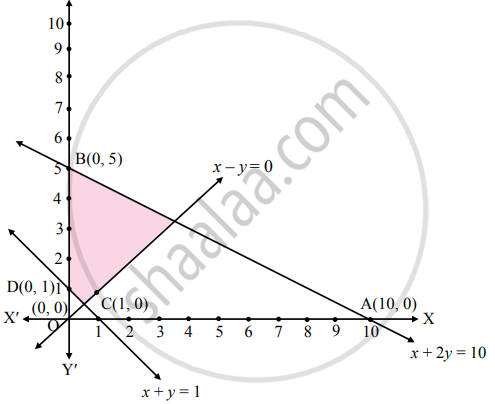# Solve the following system of inequalities graphically.x + 2y ≤ 10, x + y ≥ 1, x – y ≤ 0, x ≥ 0, y ≥ 0 - Mathematics and Statistics

Sum

Solve the following system of inequalities graphically.
x + 2y ≤ 10, x + y ≥ 1, x – y ≤ 0, x ≥ 0, y ≥ 0

#### Solution

To find graphical solution, construct the table as follows:

 Inequation Equation Double Intercept form Points (x, y) Region x + 2y ≤ 10 x + 2y = 10 "x"/10 + "y"/5 = 1 A (10, 0),B (0, 5) 0 + 2(0) ≤ 10∴ 0 ≤ 10∴ origin side x + y ≥ 1 x + y = 1 "x"/1 + "y"/1 = 1 C (1, 0),D (0, 1) 0 + 0 ≱ 1∴ 0 ≱ 1∴ non-origin side x – y ≤ 0 x – y = 0 – 0 (0, 0),E(1, 1) 0 – 0 ≤ 0∴ 0 ≤ 0∴ origin side x ≥ 0 x = 0 – – R.H.S. of Y-axis y ≥ 0 y = 0 – – Above X-axisThe shaded portion represents the graphical solution.

Concept: Graphical Solution of Linear Inequality of Two Variable
Is there an error in this question or solution?
Chapter 8: Linear Inequations - Miscellaneous Exercise 8 [Page 122]

#### APPEARS IN

Balbharati Mathematics and Statistics 2 (Commerce) 11th Standard HSC Maharashtra State Board
Chapter 8 Linear Inequations
Miscellaneous Exercise 8 | Q 15 | Page 122
Share